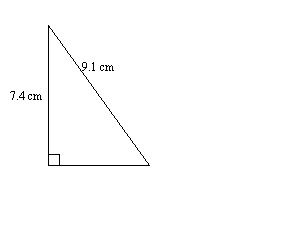Name:    Math 10W Practice Unit 5 Test #2

Multiple Choice
Identify the choice that best completes the statement or answers the question.

1.

The sine ratio relates to which two sides of a right triangle?
 a. The side opposite a given angle and the hypotenuse. b. The side adjacent to a given angle and the hypotenuse. c. The side adjacent to a given angle and the opposite side. d. The side adjacent to a given angle and the vertical side.

2.

The cosine ratio relates to which two sides of a right triangle?
 a. The side adjacent to a given angle and the vertical side. b. The side adjacent to a given angle and the hypotenuse. c. The side adjacent to a given angle and the opposite side. d. The side opposite a given angle and the hypotenuse.

3.

What is the cosine of 32°?
 a. 0.625 c. 0.848 b. 0.564 d. 0.53

4.

What is the cosine of 59°?
 a. 0.857 c. 1.664 b. 0.515 d. 0.891

5.

A right triangle has a hypotenuse of 17 cm. If one of the angles is 23°, what is the adjacent side?
 a. 7.85 cm c. 7.22 cm b. 15.65 cm d. 6.64 cm

6.

If a right triangle has a hypotenuse of 50 cm and an angle of 70°, what is the length of the side adjacent to the given angle?
 a. 46.98 cm c. 17.10 cm b. 137.37 cm d. 20.20 cm

7.

A right triangle has an angle of 15°. If the opposite side is 67 cm long, what is the length of the adjacent side?
 a. 250.0 cm c. 253.7 cm b. 258.9 cm d. 254.9 cm

8.

A right triangle has an angle of 62°. If the opposite side is 57 cm long, what is the length of the adjacent side?
 a. 34.0 cm c. 30.3 cm b. 60.6 cm d. 64.6 cm

9.

A right triangle has an angle of 50°. If the adjacent side is 28.0 cm long, what is the length of the opposite side?
 a. 39.6 cm c. 34.8 cm b. 43.6 cm d. 33.4 cm

10.

What is tan-1(0.14)?
 a. 8.05° c. 7.97° b. 39.25° d. 81.95°

1.

Solve for the unknown side length.2.

A right triangle with a hypotenuse of 25 m must have legs that are at least 3 m in length. What is the maximum length that either of the legs can be?

3.

A right triangle has a hypotenuse of 24 m. If one of the angles is 54°, what is the length of the opposite side?

4.

Gerald wants to use his kite to see if he can successfully drop a water balloon on an unsuspecting target. If his kite is flying at an angle of 70° from the ground and his target is 12 m away, how much string does Gerald need to let out on his kite?

5.

In a right triangle, the side opposite a given angle is 2.1 times larger than the adjacent side. What is the measure of the angle?